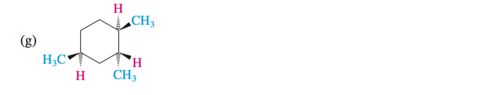# Problem: Write a structural formula for the most stable conformation of each of the following compounds:

###### FREE Expert Solution
89% (132 ratings)
###### Problem Details

Write a structural formula for the most stable conformation of each of the following compounds:Frequently Asked Questions

What scientific concept do you need to know in order to solve this problem?

Our tutors have indicated that to solve this problem you will need to apply the Equatorial Preference concept. You can view video lessons to learn Equatorial Preference. Or if you need more Equatorial Preference practice, you can also practice Equatorial Preference practice problems.

What is the difficulty of this problem?

Our tutors rated the difficulty ofWrite a structural formula for the most stable conformation ...as low difficulty.

How long does this problem take to solve?

Our expert Organic tutor, Jonathan took 1 minute and 20 seconds to solve this problem. You can follow their steps in the video explanation above.

What professor is this problem relevant for?

Based on our data, we think this problem is relevant for Professor Muller's class at University of Wisconsin - Eau Claire.

What textbook is this problem found in?

Our data indicates that this problem or a close variation was asked in Organic Chemistry - Carey 8th Edition. You can also practice Organic Chemistry - Carey 8th Edition practice problems.